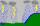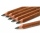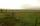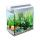# Rainfall

Annual rainfall in our country are an average of 797 mm. How many m3 of water rains on average per hectare?

Result

V =  7970 m3

#### Solution:Leave us a comment of example and its solution (i.e. if it is still somewhat unclear...):

Showing 0 comments:Be the first to comment!#### To solve this example are needed these knowledge from mathematics:

Do you want to convert area units? Do you want to convert length units?

## Next similar examples:

1. RainfallHow many liters of water did fell in a 32m long and 8m wide garden, if 8mm of rain fell?
2. One hectareHow many square meters are one hectare?
3. Argicultural fieldField has a rectangular shape with a width 180 m and circumference 940 m. How many hectares acreage has field?
4. TailorTailor has an estimated 11 meters of 80 cm wide fabric. He will shape wholly 7 rectangles measuring 80 x 150 cm and nothing fabric does not remain. How many fabric he have?
5. Percitipation - meteoDo you have any tip for calc percitipation on a lnd ? Example on 2 ha falls to 5 mm, how many cubic meters is it?
6. Bathroom 2A bathroom is 2.4 meters long and 1.8 meters wide. How many square tiles 1 dm on each side are to be used to cover it?
7. Pencil cutVeronika cut 10cm pencil. Each turn, the pencil was reduced by 0.2mm. She turned the pencil 100 times. How many centimeters has a pencil now?
8. The bookThe book has 280 pages. Each side is a rectangle with sides 15 cm and 22 cm. a) how many leaves has the book? b) at least how many square meters of paper should be used to production this book?
9. AquariumAquarium is rectangular box with square base containing 76 liters of water. Length of base edge is 42 cm. To what height the water level goes?
10. LandLand has a rectangular shape, its surface area is 1.45 hectares. Its width is 250 m. Determine the length of the land.
11. AreaCalculate: ?
12. Area of rectangleCalculate the area of rectangle in square meters whose sides have dimensions a = 80dm and b = 160dm.
13. Area of trapezoidThe trapezoid bases are and 7 dm and 11 cm. His height is 4 cm. Calculate the area of trapezoid.
14. GardenThe garden has two opposite parallel fences. Their distance is 33.1 m. Lengths in these two fences are 75.5 meters and 49.4 meters. Calculate the area of this garden.
15. Decorative fenceThe rectangular garden is 57 m long and 42 m wide. Calculate how much m² it will reduce if it encloses with a 60 cm wide decorative fence.
16. SandboxSandbox has area of 32 sq ft and length of 4 1/2 ft. What is width of sandbox.
17. Aquarium IICalculate how much glass we need to build an aquarium with a rectangular shape with base 70 cm × 70 cm and a height of 70 cm, if the waste is 2%. Aquarium haven't top glass.